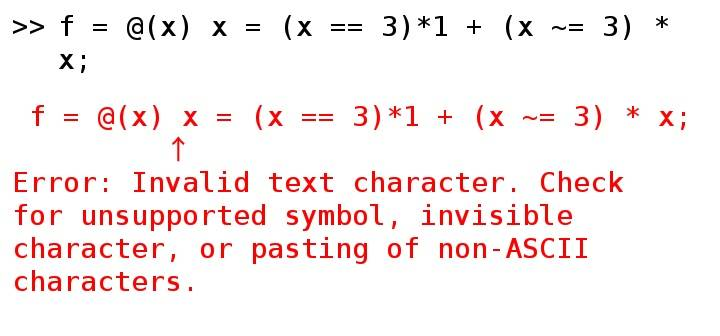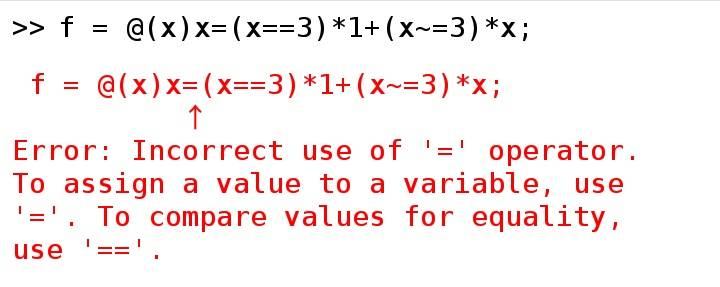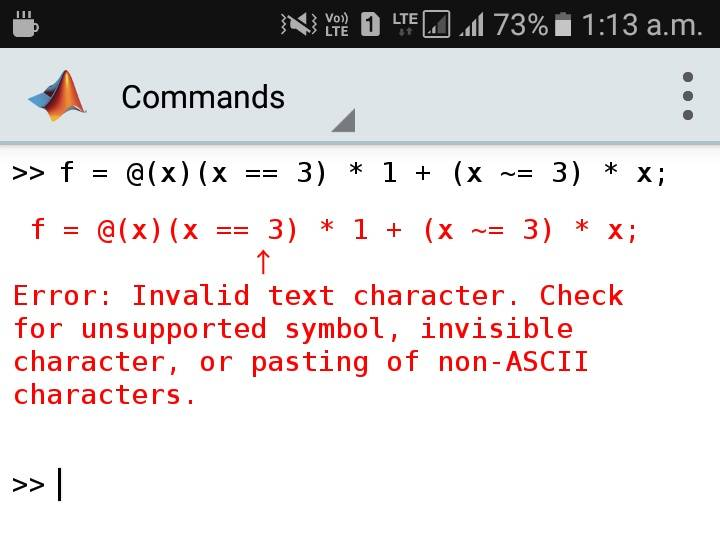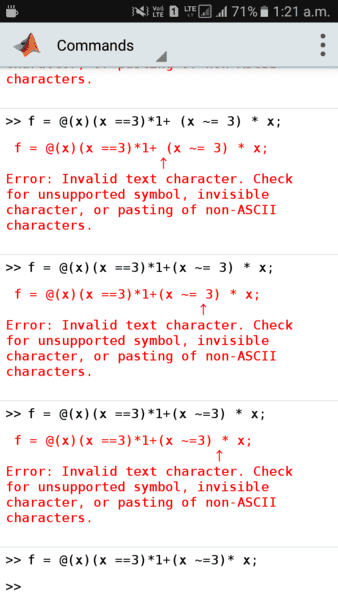# Need help defining an anonymous function in Matlab

• MATLAB
Gold Member
How can a step function be defined as an anonymous function in Matlab?
for example
for x ==3 return 1, for all other x return x.
Thanks

## Answers and Replies

Mark44
Mentor
I think this will work:
Matlab:
f = @(x) x = (x == 3)*1 + (x ~= 3) * x

f(2) should evaluate to 2 and f(3) should evaluate to 1.

I don't have matlab, so I can't test this out, but according to the MathWorks documentation on anonymous functions, it should work. The code I wrote is a bit more cumbersome than equivalent code in C/C++, since matlab doesn't have a conditional operator.

Gold Member
Thank you

Wrichik Basu
Gold Member
2020 Award
I think this will work:
Matlab:
f = @(x) x = (x == 3)*1 + (x ~= 3) * x

f(2) should evaluate to 2 and f(3) should evaluate to 1.

I don't have matlab, so I can't test this out, but according to the MathWorks documentation on anonymous functions, it should work. The code I wrote is a bit more cumbersome than equivalent code in C/C++, since matlab doesn't have a conditional operator.
I don't know if this is common for anonymous functions, but I found that whitespace is illegal, unlike java or any other programming language. In matlab mobile:Works correctly with whitespace removed.

Another thing: the "x=" part is also giving an error:Other than that, the result is coming fine.

#### Attachments

Gold Member
I don't know if this is common for anonymous functions, but I found that whitespace is illegal, unlike java or any other programming language. In matlab mobile:

View attachment 235158

Works correctly with whitespace removed.

Another thing: the "x=" part is also giving an error:

View attachment 235159

Other than that, the result is coming fine.
I appreciate your reply. Thank you. I have had the error and tried permutations trying to figure out wth. Thanks.

•Wrichik Basu
Mark44
Mentor
So, Wrichik, is this what works?
Matlab:
f = @(x)(x == 3) * 1 + (x ~= 3) * x

Gold Member
how do I mark a question answered??
Thanks

Wrichik Basu
Gold Member
2020 Award
So, Wrichik, is this what works?
Matlab:
f = @(x)(x == 3) * 1 + (x ~= 3) * x
Nope, the space is still giving an error:Could be a bug in the app. I don't have Matlab desktop version, so I cannot confirm.

#### Attachments

Mark44
Mentor
Well, that sucks... There aren't many programming languages that throw an error because you have included a space.

Wrichik Basu
Gold Member
2020 Award
Well, that sucks... There aren't many programming languages that throw an error because you have included a space.
It is quite frustrating, and I don't have an explanation. I tried all possible combinations, and this is the one (at the bottom) that was evaluated correctly (mark the spaces):#### Attachments

DrGreg
Science Advisor
Gold Member
I wonder if the "invalid text character" isn't a space but some other character that looks like a space? Or an invisible character that is there as well as a space?

Gold Member
could it be that the multiplication is attempting to be applied to a logic result.

Mark44
Mentor
I wonder if the "invalid text character" isn't a space but some other character that looks like a space? Or an invisible character that is there as well as a space?
That would be worth investigating.

could it be that the multiplication is attempting to be applied to a logic result.
I doubt this very much. In matlab, true has the value 1 and false has the value 0.

Wrichik Basu
Gold Member
2020 Award
I wonder if the "invalid text character" isn't a space but some other character that looks like a space? Or an invisible character that is there as well as a space?
That would be worth investigating.
I directly copied from here and pasted there. Should that cause any problem?

DrClaude
Mentor
I directly copied from here and pasted there. Should that cause any problem?
That is most probably the problem. Matlab has no problem with spaces (ASCII code 32), so it must be another character that is copied and pasted.

Wrichik Basu
Gold Member
2020 Award
That is most probably the problem. Matlab has no problem with spaces (ASCII code 32), so it must be another character that is copied and pasted.
You've got it right. But I copy-paste from the matlab website as well, but that doesn't create a problem. How is this possible?

Mark44
Mentor
You've got it right. But I copy-paste from the matlab website as well, but that doesn't create a problem. How is this possible?
The two web sites might be formatting text differently, with one using non-printing characters like &nbsp (non-breaking space) or similar.

I'm going to guess that my 2nd stab at it will actually work, with the extra spaces added for readability not affecting anything.
Matlab:
f = @(x) (x == 3) * 1 + (x ~= 3) * x

Last edited:
•Wrichik Basu
DrGreg
Science Advisor
Gold Member
One more thought: the text inside a "Code (Matlab M)" box on this forum is coloured, which means there is colour information as well as text. When you copy and paste into Matlab, the colour information may be corrupting the text (Rich Text?).

•Wrichik Basu